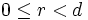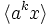# Subgroup structure of dihedral groups

This article gives specific information, namely, subgroup structure, about a family of groups, namely: dihedral group.
View subgroup structure of group families | View other specific information about dihedral group

We study here the subgroup structure of finite dihedral groups. See subgroup structure of infinite dihedral group for the subgroup structure of the infinite dihedral group.

For any natural number$n$, we define:$D_{2n} := \langle a,x \mid a^n = x^2 = e, xax^{-1} = a^{-1} \rangle$.

For$n \ge 3$,$D_{2n}$ is the group of symmetries in the regular$n$-gon in the plane.

There are two kinds of subgroups:

1. Subgroups of the form$\langle a^d \rangle$, where$d | n$. There is one such subgroup for each$d$. The total number of such subgroups is$\tau(n)$ or$\sigma_0(n)$, i.e., the number of positive divisors of$n$.
2. Subgroups of the form$\langle a^d, a^rx\rangle$ where$d | n$ and$0 \le r < d$. There are thus$d$ such subgroups for each such divisor$d$. The total number of such subgroups is$\sigma(n)$ or$\sigma_1(n)$, i.e., the sum of positive divisors of$n$.

We consider various cases when discussing subgroup structure:

• The special cases$n = 1, n = 2, n = 4$.
• The special case where$n$ is a power of$2$.
• The special case where$n$ is odd.

## The subgroup$\langle a \rangle$

Further information: Cyclic subgroup of dihedral group

### The special case$n = 1$

In the case$n = 1$, the subgroup$\langle a \rangle$ is trivial, and the whole group is cyclic of order two generated by$x$.

### The special case of$n = 2$

In the case$n = 2$, the group$D_{2n}$ is the Klein four-group:$D_4 = \langle a,x \mid a^2 = x^2 = e, ax = xa \rangle$.

For this, the subgroup$\langle a \rangle$ is a normal subgroup, but not a characteristic subgroup.

### The case$n \ge 3$

In the case$n \ge 3$, the subgroup$\langle a \rangle$ is the unique cyclic subgroup of order$n$. It satisfies the following properties:

These facts have a number of generalizations:

### The case$n \ne 1,2, 4$

In the case$n \ne 2, 4$, the subgroup$\langle a \rangle$ is the centralizer of commutator subgroup, i.e., it is the centralizer in$D_{2n}$ of the commutator subgroup of$D_{2n}$, which is$\langle a^2 \rangle$.

There are a number of generalizations/related facts:

### Odd versus even$n$

When$n$ is odd, the cyclic subgroup of order$n$ in the dihedral group of order$2n$, satisfies the following properties:

None of these properties are satisfied when$n$ is even.

Also, when$n$ is odd, the cyclic subgroup of order$n$ is the commutator subgroup.

## The subgroup$\langle x \rangle$

### General facts

• Its normalizer is$\langle a^{n/2}, x \rangle$ when$n$ is even. When$n$ is odd,$\langle x \rangle$ is self-normalizing.
• The conjugate subgroups to this subgroup are subgroups of the form$\langle a^{2r}x \rangle$. When$n$ is odd, this includes all subgroups of the form$\langle a^k x \rangle$, whereas if$n$ is even, this includes only half the subgroups of the form$\langle a^k x \rangle$. There are thus$n/2$ conjugate subgroups if$n$ is even and$n$ conjugate subgroups if$n$ is odd.
• If$n = 2$ ,there are three automorphic subgroups,$\langle a \rangle, \langle x \rangle, \langle ax \rangle$. If$n \ne 2$, the automorphic subgroups to this subgroup are subgroups of the form$\langle a^r x \rangle$. There are thus$n$ of them if$n \ne 2$.
• The normal closure of$\langle x \rangle$ is$\langle a^2, x \rangle$. This is the whole group if$n$ is odd and is a subgroup of index two if$n$ is even.

### The special case of$n = 2$

When$n = 2$, the dihedral group is the Klein four-group, and$\langle x \rangle$ is a normal subgroup. There is no other$n$ for which$\langle x \rangle$ is a normal subgroup.

### The case$n \ge 3$

Some properties satisfied whenever$n \ge 3$ are:

### Odd versus even$n$

When$n$ is odd, then the subgroup$\langle x \rangle$ satisfies the following properties:

On the other hand, when$n$ is even, this subgroup satisfies none of these properties.

### The case where$n$ is a power of two

When$n$ is a power of two, the subgroup$\langle x \rangle$ satisfies the following properties:

• Subnormal subgroup: In particular, when$n = 2^k$,$\langle x \rangle$ is a$k$-subnormal subgroup.